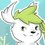# IMO 2016 Day 2

Because IMO doesn't only last for one day.

## Problem 4

A set of positive integers is called fragrant if it contains at least two elements and each of its elements has a prime factor in common with at least one of the other elements. Let $P(n) = n^2+n+1$. What is the least possible positive integer value of $b$ such that there exists a non-negative integer $a$ for which the set $\{P(a+1), P(a+2), \ldots, P(a+b)\}$ is fragrant?

## Problem 5

The equation $(x-1) (x-2) \ldots (x-2016) = (x-1) (x-2) \ldots (x-2016)$ is written on the board, with 2016 linear factors on each side. What is the least possible value of $k$ for which it is possible to erase exactly $k$ of these 4032 linear factors so that at least one factor remains on each side and the resulting equation has no real solutions?

## Problem 6

There are $n \ge 2$ line segments in the plane such that every two segments cross and no three segments meet at a point. Geoff has to choose an endpoint of each segment and place a frog on it facing the other endpoint. Then he will clap his hands $n-1$ times. Every time he claps, each frog will immediately jump forward to the next intersection point on its segment. Frogs never change the direction of their jumps. Geoff wishes to place the frogs in such a way that no two of them will every occupy the same intersection point at the same time.

• (a) Prove that Geoff can always fulfill his wish if $n$ is odd.
• (b) Prove that Geoff can never fulfill his wish if $n$ is even.Note by Ivan Koswara
5 years, 2 months ago

This discussion board is a place to discuss our Daily Challenges and the math and science related to those challenges. Explanations are more than just a solution — they should explain the steps and thinking strategies that you used to obtain the solution. Comments should further the discussion of math and science.

When posting on Brilliant:

• Use the emojis to react to an explanation, whether you're congratulating a job well done , or just really confused .
• Ask specific questions about the challenge or the steps in somebody's explanation. Well-posed questions can add a lot to the discussion, but posting "I don't understand!" doesn't help anyone.
• Try to contribute something new to the discussion, whether it is an extension, generalization or other idea related to the challenge.

MarkdownAppears as
*italics* or _italics_ italics
**bold** or __bold__ bold
- bulleted- list
• bulleted
• list
1. numbered2. list
1. numbered
2. list
Note: you must add a full line of space before and after lists for them to show up correctly
paragraph 1paragraph 2

paragraph 1

paragraph 2

[example link](https://brilliant.org)example link
> This is a quote
This is a quote
    # I indented these lines
# 4 spaces, and now they show
# up as a code block.

print "hello world"
# I indented these lines
# 4 spaces, and now they show
# up as a code block.

print "hello world"
MathAppears as
Remember to wrap math in $$ ... $$ or $ ... $ to ensure proper formatting.
2 \times 3 $2 \times 3$
2^{34} $2^{34}$
a_{i-1} $a_{i-1}$
\frac{2}{3} $\frac{2}{3}$
\sqrt{2} $\sqrt{2}$
\sum_{i=1}^3 $\sum_{i=1}^3$
\sin \theta $\sin \theta$
\boxed{123} $\boxed{123}$

Sort by:

@Geoff Pilling Congrats. You've been featured in the IMO.

- 5 years, 2 months ago

Hahaha... Woo hoo! Thanks!

Geoff

- 5 years, 2 months ago

Typo in problem 2

- 5 years, 2 months ago